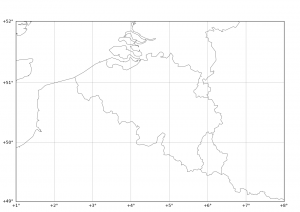# Matplotlib Basemap tutorial 01 : Your first map```#
# BaseMap example by geophysique.be
# tutorial 01

from mpl_toolkits.basemap import Basemap
import matplotlib.pyplot as plt
import numpy as np
fig = plt.figure(figsize=(11.7,8.3))
#Custom adjust of the subplots
plt.subplots_adjust(left=0.05,right=0.95,top=0.90,bottom=0.05,wspace=0.15,hspace=0.05)
ax = plt.subplot(111)
#Let's create a basemap around Belgium
m = Basemap(resolution='i',projection='merc', llcrnrlat=49.0,urcrnrlat=52.0,llcrnrlon=1.,urcrnrlon=8.0,lat_ts=51.0)
m.drawcountries(linewidth=0.5)
m.drawcoastlines(linewidth=0.5)

m.drawparallels(np.arange(49.,53.,1.),labels=[1,0,0,0],color='black',dashes=[1,0],labelstyle='+/-',linewidth=0.2) # draw parallels
m.drawmeridians(np.arange(1.,9.,1.),labels=[0,0,0,1],color='black',dashes=[1,0],labelstyle='+/-',linewidth=0.2) # draw meridians

plt.show()```

## 5 thoughts on “Matplotlib Basemap tutorial 01 : Your first map”

1.max says:

Hi

that script is missing a “import numpy as np”

Adding this, it works fine

thanks for your helpful website!
max

2.Thomas Lecocq says:

Indeed ! Thanks, it’s corrected !

3.Takeshi says:

Hi,

With learning the textbook “Python for Data Analysis” by Wes McKinney, I found your blog. It has a plenty of good tips about basemap programming. Thanks.

Please let me ask a question. I tried the code above, but my IDE returned just “”. When I removed the dashes options (dashes=[1,0]) in m.drawparallels and m. drawmeridians, the code got worked. I’m not sure what is going on. It might be some conflict due to package environment.

Regards,
Takeshi

4.Thomas Lecocq says:

Hi !

Thanks for using my blog!

Actually, I am not sure what could be the problem… What Python are you using ? 2.7.x ? Anaconda ?

Cheers

Thomas

5.mark says:

Hello Thomas,
thanks for your tutorial. I just wanted to note that I had the same problem that Takeshi noted in his comment above… When I removed the dashes=[1,0] from m.drawparallels and m.drawmeridians, it worked fine. FYI – I’m using Python 2.7 with Anaconda.
I’m not looking for a response… I just thought you may be interested.

Thank you again.
Mark